# demos_source/code_TestPasson.c File Reference

`#include "femtoos_code.h"`

Include dependency graph for code_TestPasson.c: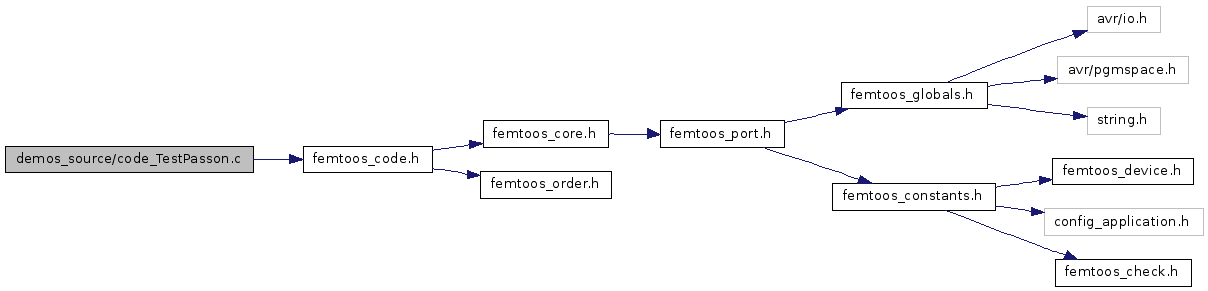Go to the source code of this file.

## Defines

This file is solely for demonstration purposes.
#define setLed(lednr, state)   if (state) { devLedPORT |= (1 << lednr); } else { devLedPORT &= ~(1 << lednr); }

## Functions

void appBoot (void)
Prototype for the general initialization.
static void HandOver (Tuint08 uiLed) __attribute__((naked
void appLoop_Reset (void)
void appLoop_AutoStart (void)

## Variables

static void noreturn
static void always_inline

## Define Documentation

 #define setLed ( lednr, state ) if (state) { devLedPORT |= (1 << lednr); } else { devLedPORT &= ~(1 << lednr); }

Definition at line 53 of file code_TestPasson.c.

This file is solely for demonstration purposes.

This demo is use to demonstrate the power of mutexes and reentrant behaviour. Every led gets a state (on/off) from its right neighbour and tries to pass that on to the left. The last led (to the left) is coupled to the led on the right, so the form a circle. Every 30 seconds a seed is given to the right most led to get the processes running.

Definition at line 41 of file code_TestPasson.c.

Referenced by appLoop_AutoStart(), appLoop_Reset(), and HandOver().

Definition at line 78 of file code_TestPasson.c.

Referenced by appLoop_AutoStart(), appLoop_Reset(), and HandOver().

## Function Documentation

 void appBoot ( void )

Prototype for the general initialization.

This example is made to demonstrate how the tick hooks can be used. There are three tick hooks present. One on every tick, one on every tick block (256 ticks) and one on every rollover (65536 ticks). They all are coupled to a counter and with one switch you can switch between the different hooks. The counter is display on the ledport. (PORTA on the ATtiny861)

Definition at line 46 of file code_TestPasson.c.

 void appLoop_AutoStart ( void )

Definition at line 178 of file code_TestPasson.c.

Here is the call graph for this function:Definition at line 118 of file code_TestPasson.c.

References HandOver().

Here is the call graph for this function:Definition at line 122 of file code_TestPasson.c.

References HandOver().

Here is the call graph for this function: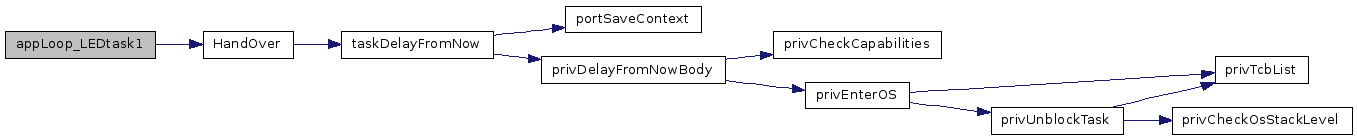Definition at line 126 of file code_TestPasson.c.

References HandOver().

Here is the call graph for this function: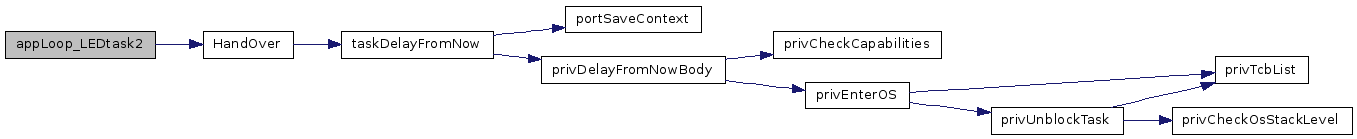Definition at line 130 of file code_TestPasson.c.

References HandOver().

Here is the call graph for this function: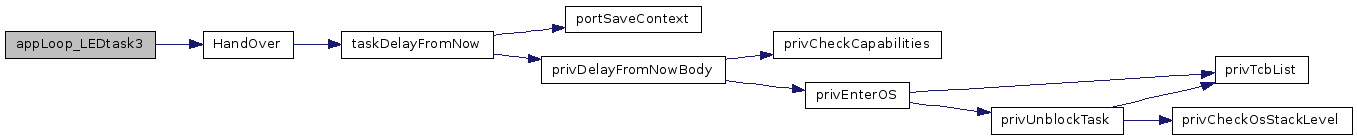Definition at line 134 of file code_TestPasson.c.

References HandOver().

Here is the call graph for this function: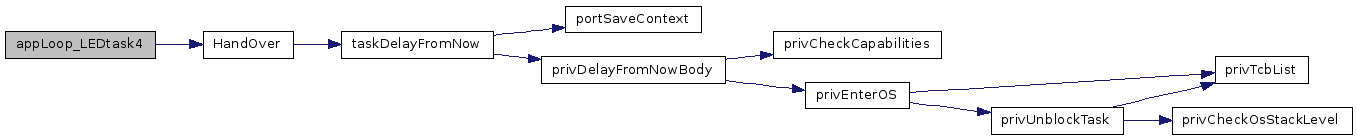Definition at line 138 of file code_TestPasson.c.

References HandOver().

Here is the call graph for this function:Definition at line 142 of file code_TestPasson.c.

References HandOver().

Here is the call graph for this function:Definition at line 146 of file code_TestPasson.c.

References HandOver().

Here is the call graph for this function:void appLoop_Reset ( void )

Definition at line 157 of file code_TestPasson.c.

Here is the call graph for this function: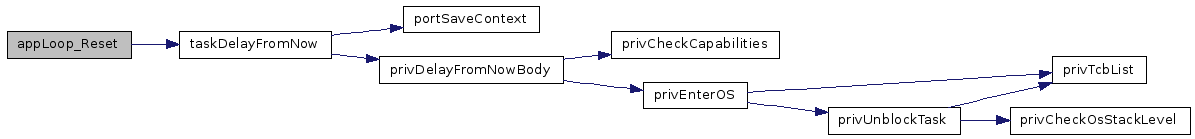static void HandOver ( Tuint08 uiLed ) ` [static]`

Definition at line 80 of file code_TestPasson.c.

Here is the call graph for this function:Here is the caller graph for this function: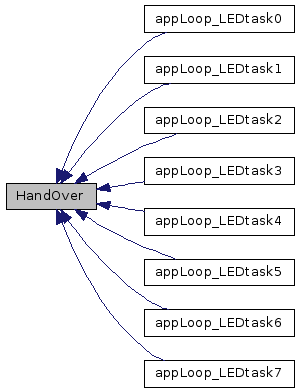## Variable Documentation

 void always_inline

Definition at line 58 of file code_TestPasson.c.

 void noreturn

Definition at line 58 of file code_TestPasson.c.

Generated on Fri Oct 16 00:05:24 2009 for FemtoOS by1.5.2